• 表达式2*(9+6/3-5)+4，称为中缀表达式，表示成2 9 6 3 / + 5 - * 4 +称为后缀表达式，表示成+ * 2 - + 9 / 6 3 5 4称为前缀表达式。 ·基本要求 将中缀表达式，转换为后缀表达式和前缀表达式，再分别计算转换后的...
• 该程序实现了运算表达式转换为中缀表达式中缀表达式转换为后缀表达式及后缀表达式求值。该程序已实现加减乘除括号运算符及求余、幂指数的求解
• 中缀表达式前缀表达式求值 首先将中缀表达式转换成前缀表达式 前缀表达式中，操作符在前 例如：1+2*(5-3)+4 后缀表达式：++1*2-534 一、转换思路 转换思路为将输入的中缀表达式字符串从右往左扫描(字符前后加#号)...

中缀表达式转前缀表达式求值

首先将中缀表达式转换成前缀表达式
前缀表达式中，操作符在前
例如：1+2*(5-3)+4
后缀表达式：++1*2-534

一、转换思路

转换思路为将输入的中缀表达式字符串从右往左扫描(字符前后加#号)，遇到数字直接输出，遇到操作符比较优先级。
栈顶优先级低，入栈；
栈顶优先级高，出栈并且输出；
优先级相等(即左右括号)，出栈(不输出)；
当扫描的字符为#并且栈顶字符为#时，此时将输出的字符序列反转即为前缀表达式。
注意：优先级顺序表与求中缀表达式和后缀表达式的优先级顺序表不同

二、前缀表达式求值过程

将转换成的前缀表达式的字符串从右往左扫描，扫描到数字时进栈，扫描到字符从栈中出两个数字，然后与扫描到的字符之间进行运算， <运算时注意：先出栈的做运算符的前操作数，后出栈的做后操作数> 这与后缀、前缀表达式求值恰好相反。当全部扫描完后，栈顶的元素即为运算结果。

前缀表达式求值优先级：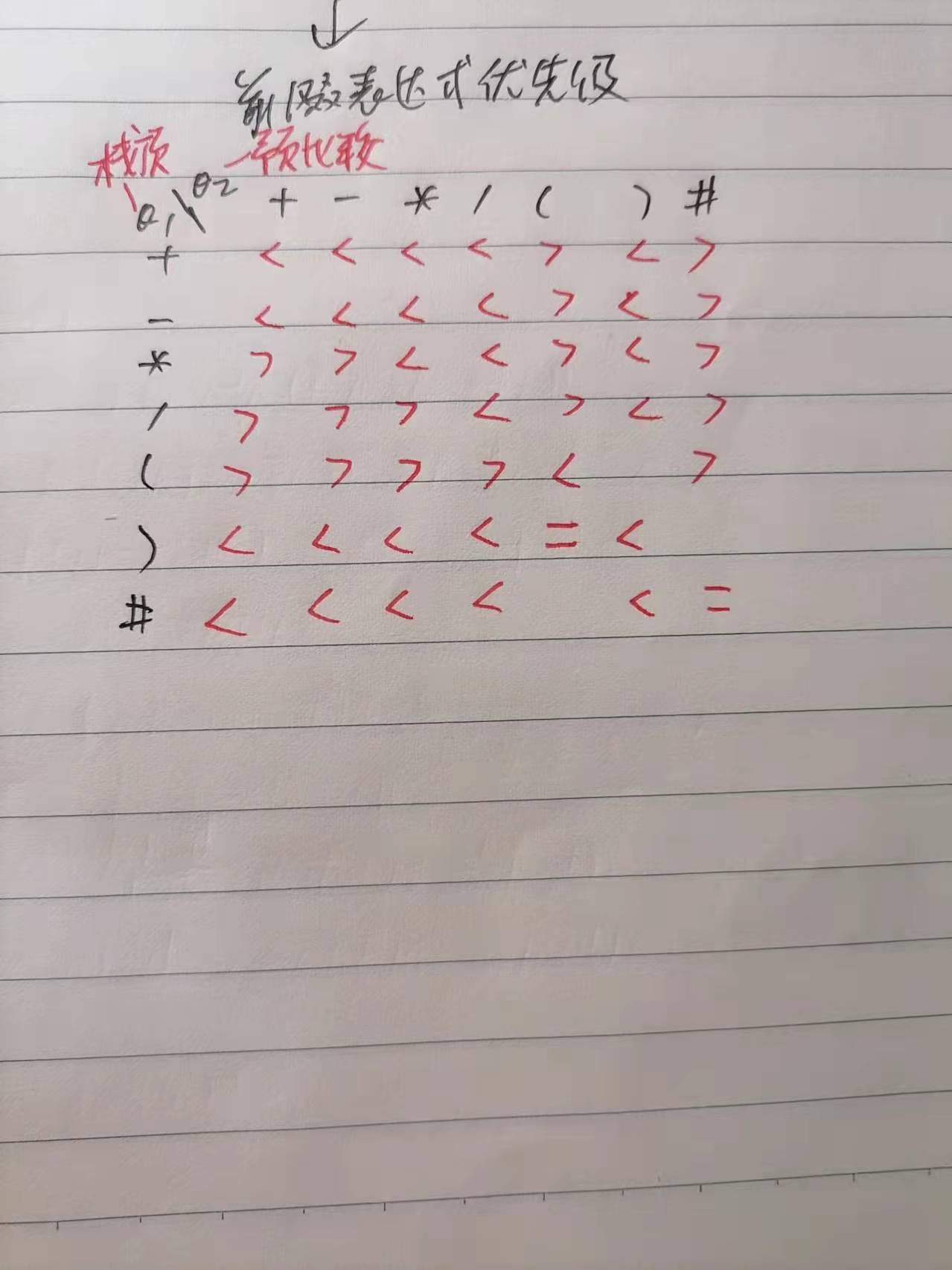该优先级表与中缀和后缀表达式求值区别主要有：相同操作符(+ - * /)栈顶优先级低；预比较运算符 ） 优先级最高，（ 最低栈顶 )、 预比较 ( 相等

三、代码展示

#include <iostream>
#include <string>
#include <algorithm>
using namespace std;

string s;  //中缀表达式字符串
string s1="\0"; //后缀表达式字符串
char OPTR; //操作符栈
char OPND; //操作数栈
int topN=-1,topT=-1;

//栈的基本操作
void push(char *OPTR,char ch){
OPTR[++topT]=ch;
}
void push(int *OPND,int val){
OPND[++topN]=val;
}
char pop(char *OPTR){
return OPTR[topT--];
}
int pop(int *OPND){
return OPND[topN--];
}
int getTop(int *OPND){
return OPND[topN];
}
char getTop(char *OPTR){
return OPTR[topT];
}

//定义一个二位字符数组存放优先级表
// +   -    *   /   (   )   #
char arr={'<','<','<','<','>','<','>',
'<','<','<','<','>','<','>',
'>','>','<','<','>','<','>',
'>','>','>','<','>','<','>',
'>','>','>','>','<','\0','>',
'<','<','<','<','=','<','\0',
'<','<','<','<','\0','<','='
};
//定义字符表
int opCH(char ch){
int i;
switch(ch){
case '+':{
i=0;
break;
}
case '-':{
i=1;
break;
}
case '*':{
i=2;
break;
}
case '/':{
i=3;
break;
}
case '(':{
i=4;
break;
}
case ')':{
i=5;
break;
}
case '#':{
i=6;
break;
}
}
return i;
}
//比较运算符的优先级
char percede(char ch1,char ch2){
int i,j;
i=opCH(ch1);
j=opCH(ch2);
return arr[i][j];
}

int operate(int a,char theta,int b){
switch(theta){
case '+':{
return a+b;
}
case '-':{
return a-b;
}
case '*':{
return a*b;
}
case '/':{
return a/b;
}
}
return 0;

}
void suffix(string s){
//将字符串反转 然后在字符串最后加一个#标志符
reverse(s.begin(),s.end());
s+="#";
for(int i=0;s[i]!='#'||getTop(OPTR)!='#';i++){
if(s[i]>48&&s[i]<58){
s1+=s[i];  //如果是数字的话放入新字符串
}else if(s[i]=='*'||s[i]=='/'||s[i]=='+'||s[i]=='-'||
s[i]=='('||s[i]==')'||s[i]=='#'){
switch(percede(getTop(OPTR),s[i])){
case '>':{
s1+=pop(OPTR);//栈顶优先级高的话放入新字符串
i--;
break;
}
case '<':{
push(OPTR,s[i]);
break;
}
case '=':{
if(s[i]=='('){
pop(OPTR);
}
}
}
}
}
}

int calculation(string s1){
int a,b,c;
char theta;
reverse(s1.begin(),s1.end());
for(int i=0;s1[i]!='\0';i++){
if(s1[i]>48&&s1[i]<58){
c=s1[i]-48;
push(OPND,c);
}else if(s1[i]=='*'||s1[i]=='/'||s1[i]=='+'||s1[i]=='-'){
a=pop(OPND);
b=pop(OPND);
theta=s1[i];
push(OPND,operate(a,theta,b));

}
}
return getTop(OPND);
}
int main(){
push(OPTR,'#');
cout<<"请输入中缀表达式:";
cin>>s;
suffix(s);
cout<<"前缀表达式：";
//反转字符串
reverse(s1.begin(),s1.end());
cout<<s1<<endl;
cout<<"表达式结果：";
cout<<calculation(s1)<<endl;
return 0;
}

四、结果展示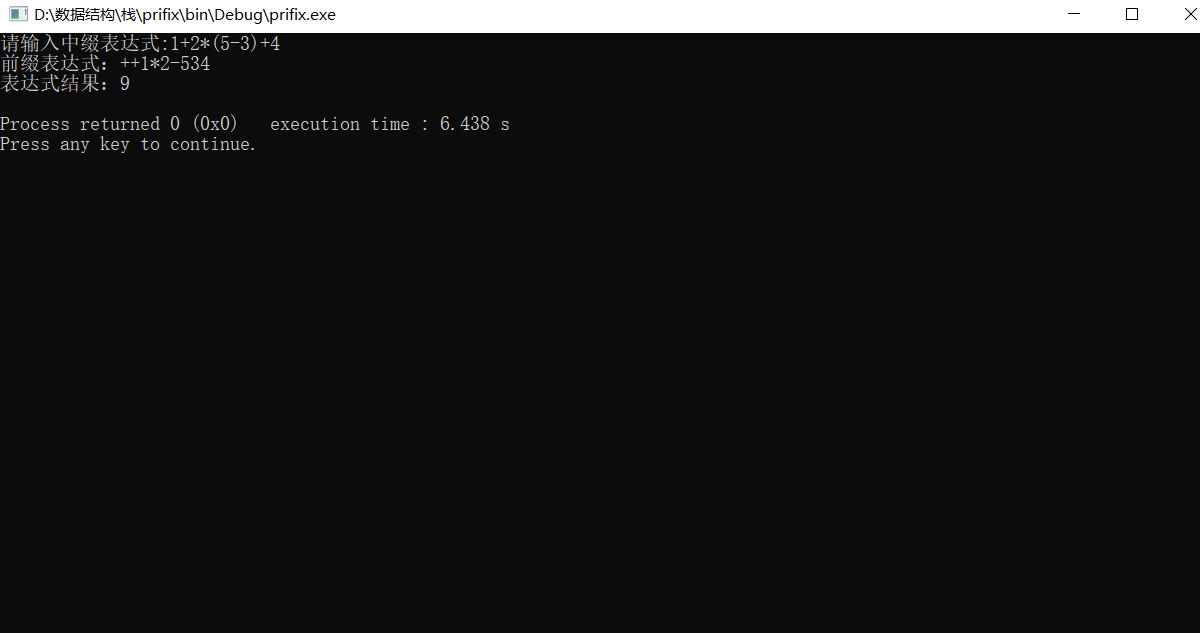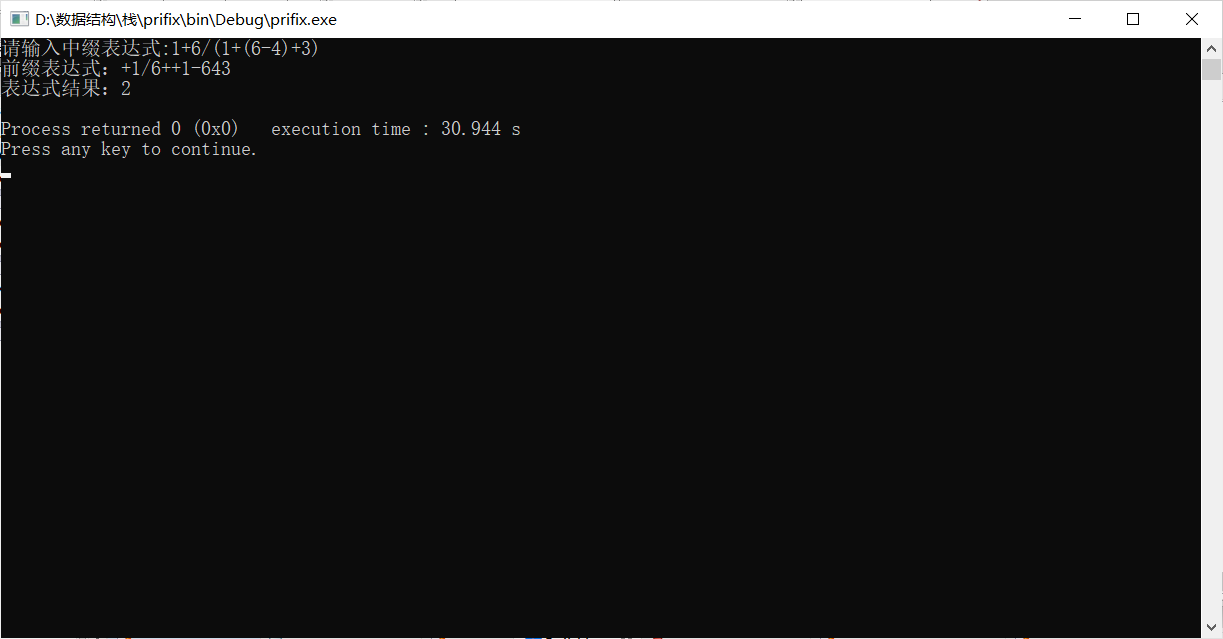五、局限

局限输入、中间或者结果值只能在0-9范围内，因为ASCII码转数字的问题只能是0-9

展开全文数据结构 算法
• 中缀表达式 转 前缀表达式

千次阅读 2021-03-30 16:48:48
接着说 中缀表达式前缀表达式 原理相同 中缀表达式: (6+3*(7-4))-8/2 1.直接转换法 确定表达式的运算方式, 加括号, 给每一次能运算的都加上: (6+(3*(7-4)))-8/2 (6+(3*(7-4)))-(8/2) ((6+(3*(7-4)))-(8/2)) ...

上一篇文章讲述了 中缀表达式 转成 后缀表达式

接着说 中缀表达式 转 前缀表达式

原理相同
中缀表达式: (6+3*(7-4))-8/2

1.直接转换法
1. 确定表达式的运算方式, 加括号, 给每一次能运算的都加上:
• (6+(3*(7-4)))-8/2
• (6+(3*(7-4)))-(8/2)
• ((6+(3*(7-4)))-(8/2))
1. 从最里面的一层括号开始运算，转换成后缀表达式的方法为：（忽略括号）符号在前，数字在后
• (7-4) => -74
• (3*(7-4)) => (3*(-74)) => *3-74 (把-74看成一个整体)
• (6+(3*(7-4))) => (6+ (*3-74 )) => +6 3-74 (把3-74看成一个整体)
• (8/2) => /82
• ((6+(3*(7-4)))-(8/2)) => (+6 *3-74 ) - ( /82 ) => - +6 *3-74 /82 (把(+6 *3-74 ) 和 ( /82)) 看成一个整体
2.利用栈
以下来自百度百科:
• (1) 首先构造一个运算符栈(也可放置括号)，运算符(以括号为分界点)在栈内遵循越往栈顶优先级不降低的原则进行排列。
• (2)从右至左扫描中缀表达式，从右边第一个字符开始判断：
如果当前字符是数字，则分析到数字串的结尾并将数字串直接输出。
如果是运算符，则比较优先级。如果当前运算符的优先级大于等于栈顶运算符的优先级(当栈顶是括号时，直接入栈)，则将运算符直接入栈；否则将栈顶运算符出栈并输出，直到当前运算符的优先级大于等于栈顶运算符的优先级(当栈顶是括号时，直接入栈)，再将当前运算符入栈。
如果是括号，则根据括号的方向进行处理。如果是向右的括号，则直接入栈；否则，遇向左的括号前将所有的运算符全部出栈并输出，遇右括号后将向左、向右的两括号一起出栈(并不输出)。
• (3) 重复上述操作(2)直至扫描结束，将栈内剩余运算符全部出栈并输出，再逆缀输出字符串。中缀表达式也就转换为前缀表达式了。
– 如果表达式结束，但栈中还有元素，将所有元素出栈，添加到前缀表达式中

可能👀看文字有点晕, 直接上图

中缀表达式: (6+3*(7-4))-8/2

1. 首先分配2个栈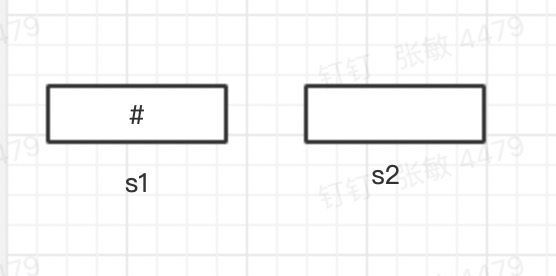1. 取字符
• 2 运算符 直接入 s2; / 操作符 入 s1;
• 8运算符 入 s2;
• ’ -'操作符 入 s1; 栈顶元素 / 优先级大于 - 所以取出/ 入s2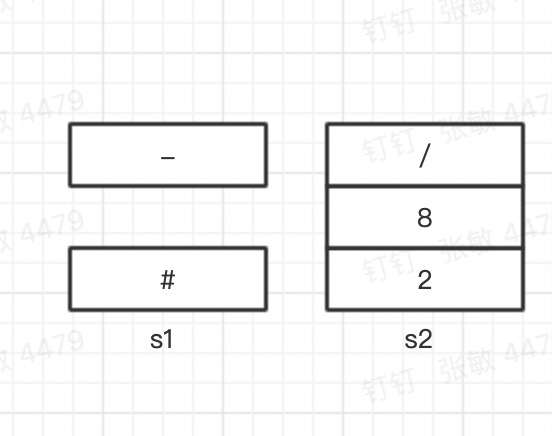- )操作符 入 s1;
-  )操作符 入 s1;
-  4 运算符 入 s2;
- '- 操作符 入 s1;
-  7运算符 入 s2;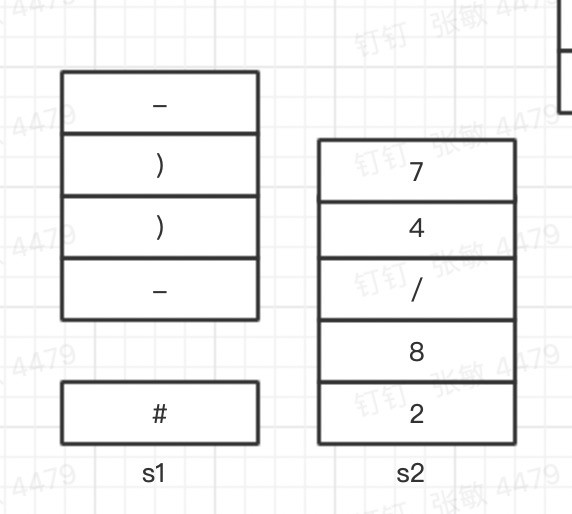• (操作符 将- 放入 s2 中, 移除 ( ) 两个;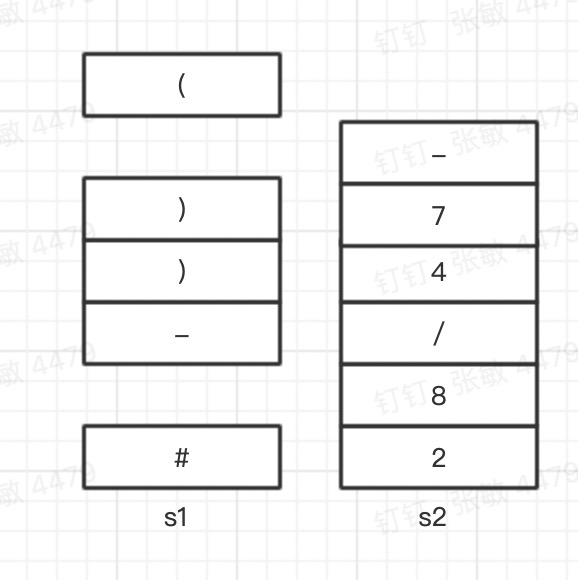• *操作符 入 s1;

• 3 运算符 入 s2;

• '+ 操作符 , 入s1, 但是 栈顶* 优先级大于 + 所以 * 出栈 入 s2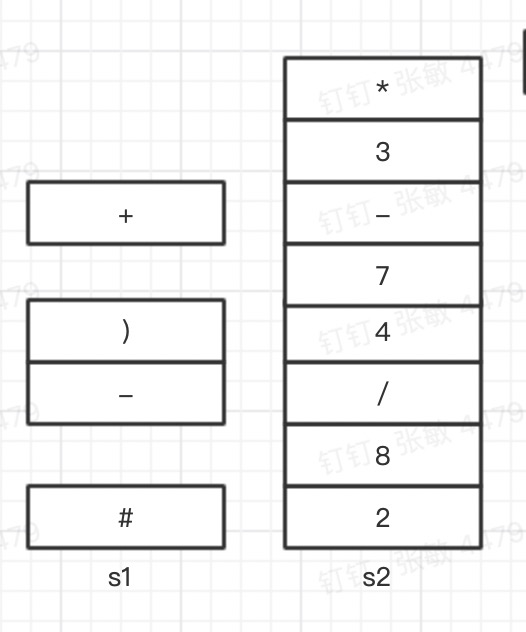• 6 运算符 入 s2;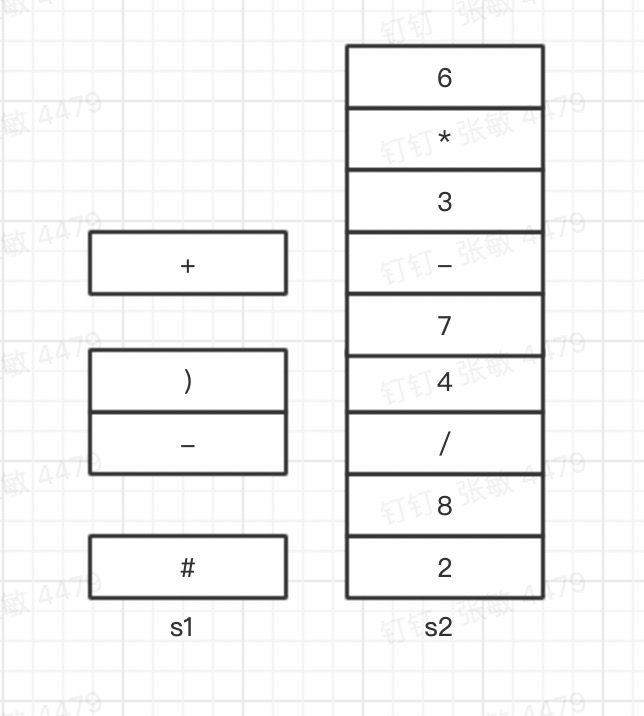• ( 取出 + 作废 ) ( ;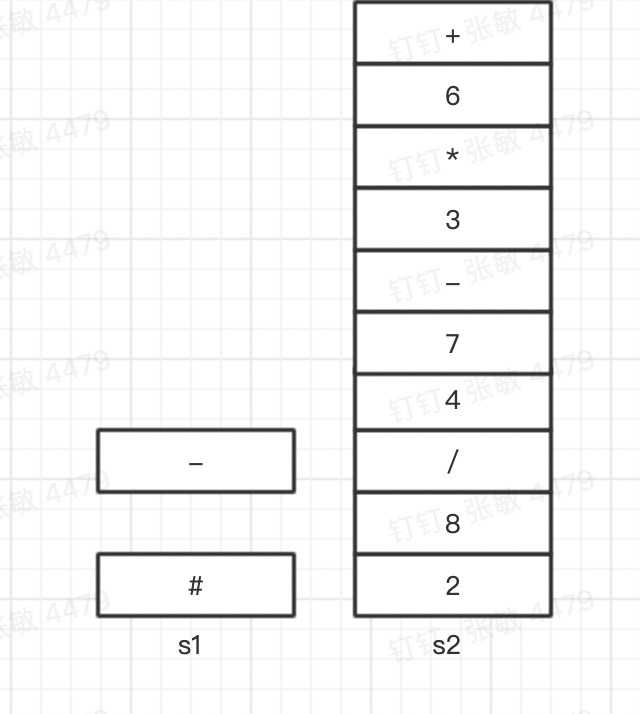• 结束 依次取出s1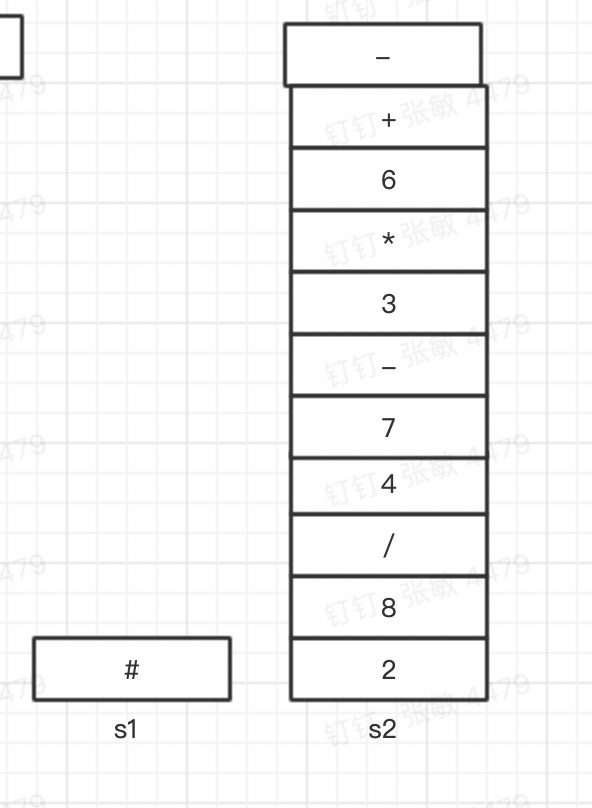逆缀 输出 - + 6 * 3-74/82

展开全文前缀表达式 算法 数据结构
• 2、利用栈实现中缀表达式前缀表达式的转换。 三、相关内容介绍 标准的表达式如"A+B"，在数学上学名叫中缀表达式（Infix Notation），原因是运算符号在两个运算对象的中间。相对应的还有前缀表达式（Prefix ...
• //用于指向字符串(中缀表达式)的指针 //声明两个栈，一个float型，一个char型 //表达式中操作数存在一个栈内，运算符存入一个栈内 //top1,top2用于指向两个栈的栈顶 float s1[maxSize];int top1=-1; char ...
#include<stdio.h>
#include<stdlib.h>
#include<string.h>
#include<math.h>
#define Min 1e-8
#define maxSize 10
int priority(char p){
if(p=='+'||p=='-'){
return 0;
}else{
return 1;
}
}
//子表达的计算
int calSub(float opand1,char op,float opand2,float &result){
//根据操作符进行计算
if(op == '*') result=opand1*opand2;
if(op == '+') result=opand1+opand2;
if(op == '-') result=opand1-opand2;
if(op == '/'){//若是除则需要判断除数为零的情况
if(fabs(opand2)<Min){// 浮点数没有准确的表达，只能用绝对值来表示差不多的范围
return 0;
}
else{
result =opand1/opand2;
}
}
return 1;
}
int calStackTopTwo(float s1[],int &top1,char s2[],int &top2){
float a=s1[top1--];//弹出栈顶元素后指针下移
float b=s1[top1--];
float result;
char op=s2[top2--];
int flag=calSub(b,op,a,result);//进行子表达式的计算
if(flag==0){
printf("被除数为零");
return 0;
}
s1[++top1]=result;//计算结果存入栈顶
return 1;

}
//计算表达式的函数
float getResult(char exp[]){
int i=0;//用于指向字符串(中缀表达式)的指针
//声明两个栈，一个float型，一个char型
//表达式中操作数存在一个栈内，运算符存入一个栈内
//top1,top2用于指向两个栈的栈顶
float s1[maxSize];int top1=-1;
char s2[maxSize];int top2=-1;

while(exp[i]!='\0'){//字符串结尾以'\0'
if(exp[i]>='0'&&exp[i]<='9'){//判断是否是数字，但此处只能判断0-9之间的一位数字
s1[++top1]=exp[i]-'0';//字符串转换为数字
++i;//下一个字符
}
else if(exp[i]=='(') {//如果是左括号的话直接进栈
s2[++top2]='(';
i++;
}else if(exp[i]=='*'|| //如果是运算符则进行以下判断
exp[i]=='/'||
exp[i]=='+'||
exp[i]=='-'){
//如果栈顶为左括号或者栈空，或者当前符号优先级大于栈顶符号优先级则入栈
if(s2[top2]=='('||top2==-1||priority(exp[i])>priority(s2[top2])){
s2[++top2]=exp[i];
i++;
}else{
int flag=calStackTopTwo(s1,top1,s2,top2);//当前运算符小于栈顶元素优先级则弹出数据栈的两个
if(flag==0){
return 0;
}
}                                  //操作数，进行运算后存入栈顶，完毕后i的值不发生
}                                      //变化
else if(exp[i]==')'){//如果碰到右括号，则弹出栈内直至左括号的所有运算符
while(s2[top2]!='('){//弹出一个运算符都需要进行计算
int flag=calStackTopTwo(s1,top1,s2,top2);
if(flag==0){
return 0;
}

}
--top2;//更新栈顶指针
i++;

}

}
//当表达式进栈完毕后
//只要符号栈内还有元素就继续进行运算
while(top2!=-1){
int flag=calStackTopTwo(s1,top1,s2,top2);
if(flag==0){
return 0;
}
}
return s1[top1];
}
//后缀表达式计算
float calPostFix(char exp[]){
float s1[maxSize];
int top=-1;
int i=0;
while(exp[i]!='\0'){
if(exp[i]>='0'&&exp[i]<='9'){
s1[++top]=exp[i]-'0';
}else{
float a,b,result;
a=s1[top--];
b=s1[top--];//出栈顶的两个元素
int flag=calSub(a,exp[i],b,result);//子表达式计算结果存入result
if(flag==0){
printf("Error");
return 0;
}
s1[++top]=result;//将结果存入栈顶
}
i++;

}
return s1[top];

}
float calPreFix(char exp[],int length){
float s1[maxSize];
int top=-1;
for(int i=length-1;i>=0;--i){
if(exp[i] >= '0' && exp[i]<='9'){
//printf("%c",exp[i]);
s1[++top]=exp[i]-'0';
}else
{
float a,b,result;
a=s1[top--];
b=s1[top--];//出栈顶的两个元素
//	printf("%f %f",s1,s1);
int flag=calSub(a,exp[i],b,result);//子表达式计算结果存入result
if(flag==0){
printf("Error");
return 0;
}
s1[++top]=result;//将结果存入栈顶
}
}
return s1[top];
}
//中缀式转后缀式
//参数top传引用型是为了能够查看栈的最大用量
//参数s2[]数组是存储结果表达式
void infixtoPostFix(char infix[],char s2[],int &top2){
char s1[maxSize];//辅助栈
int i=0,top1=-1;
while(infix[i]!='\0'){

if(infix[i]<='9'&&infix[i]>='0'){
s2[++top2]=infix[i];
i++;
}else if(infix[i]=='('){
s1[++top1]=infix[i];

i++;
}else if(infix[i]=='*'||infix[i]=='+'||infix[i]=='-'||infix[i]=='/'){
if(top1==-1||s1[top1]=='('||priority(infix[i])>priority(s1[top1])){
//中缀转后缀，当前运算符优先级小于等于栈顶运算符优先级则出栈
s1[++top1]=infix[i];
i++;
}else{
s2[++top2]=s1[top1--];
}
}else if(infix[i]==')'){
while(s1[top1]!='('){
s2[++top2]=s1[top1--];

}
--top1;//
i++;
}
}
while(top1!=-1){
s2[++top2]=s1[top1--];

}
}
//中缀式转前缀式
void infixtoPreFix(char infix[],int length,char s3[],int &top2){
char s1[maxSize];//辅助栈
char s2[maxSize];//
int i=length-1,top1=-1;
while(i>=0){
if(infix[i]<='9'&&infix[i]>='0'){
s2[++top2]=infix[i];
i--;
}else if(infix[i]==')'){
s1[++top1]=infix[i];
i--;
}else if(infix[i]=='*'||infix[i]=='+'||infix[i]=='-'||infix[i]=='/'){
//中缀转前缀，当前运算符优先级小于栈顶运算符优先级则出栈
if(top1==-1||s1[top1]==')'||priority(infix[i]) >= priority(s1[top1])){
s1[++top1]=infix[i];
i--;
}else{
s2[++top2]=s1[top1--];
}
}else if(infix[i]=='('){
while(s1[top1]!=')'){
s2[++top2]=s1[top1--];
}
--top1;
i--;
}
}
while(top1!= -1){
s2[++top2]=s1[top1--];
}
int v=0;
for(int i=top2;i>=0;i--){

s3[v++]=s2[i];
}

}
int getlength(char s[]){
int length=0;
for(int i=0;i<maxSize;i++){
if((s[i]<'9'&&s[i]>'0')||s[i]=='+'||s[i]=='*'||
s[i]=='/'||s[i]=='-'||s[i]=='('||s[i]==')'){
length++;
}
}
return length;
}
int main(){
char s[maxSize];
printf("请输入中缀表达式：\n");
scanf("%s",s);//录入字符串
printf("%s=%f\n",s,getResult(s));

char result[maxSize];//存放转换后结果
int top1=-1;
infixtoPostFix(s,result,top1);
printf("中缀转后缀为：%s\n",result);
printf("后缀的结果表达式为：%f\n",calPostFix(result));
int top2=-1;
char result1[maxSize];//存放转换后结果

infixtoPreFix(s,getlength(s),result1,top2);
printf("中缀转前缀为：%s\n",result1);
printf("前缀的结果表达式为：%f\n",calPreFix(result1,getlength(s)));

}

代码运行截图：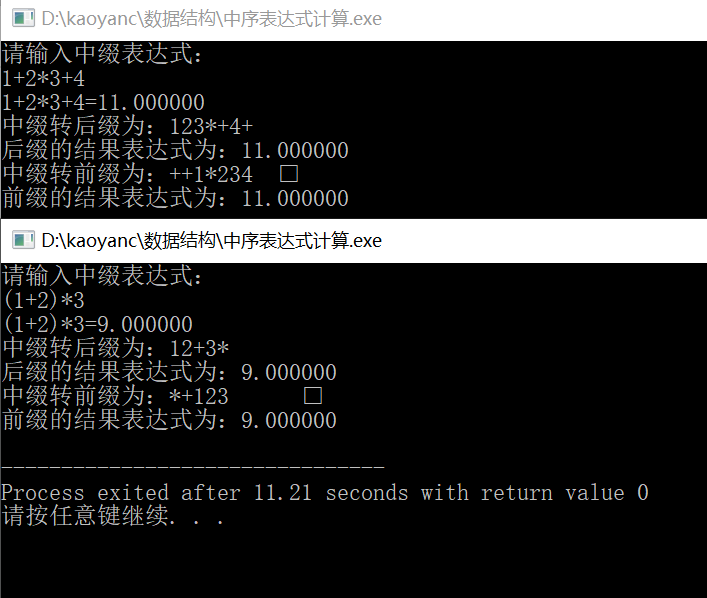其中在转化时需要注意：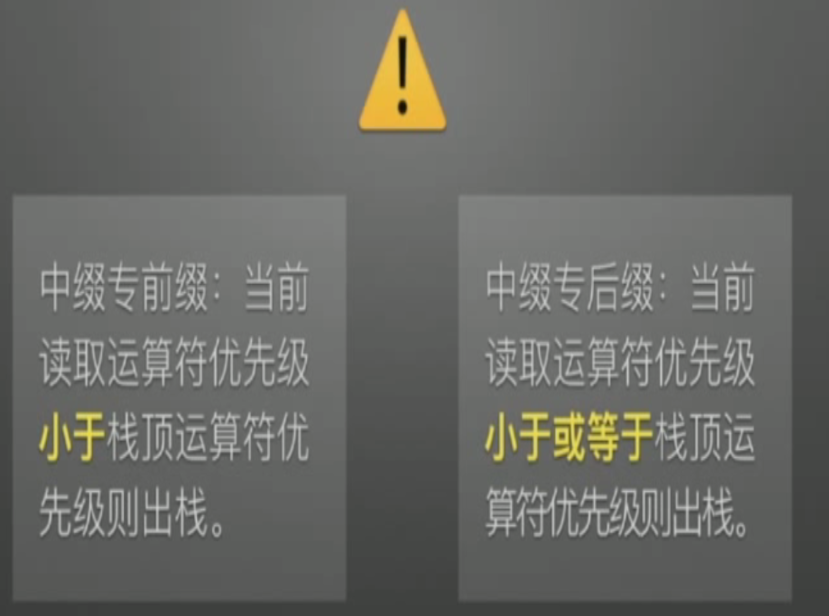展开全文• 举例：中缀表达式求后缀表达式 a+b*(c-d)-e/f 根据运算符的优先级给表达式加上括号 ((a+(b*(c-d)))-(e/f)) 将最外层的符号先提取到对应括号的右侧：（前缀表达式与之相反，是将运算符移动到对应括号的左侧，...

一、根据运算符的优先级加括号法

举例：中缀表达式求后缀表达式

a+b*(c-d)-e/f

1. 根据运算符的优先级给表达式加上括号           ((a+(b*(c-d)))-(e/f))
2.   将最外层的符号先提取到对应括号的右侧：（前缀表达式与之相反，是将运算符移动到对应括号的左侧，不再举例
• （（a+（b*（c-d）））（e/f）） ）  //相对整个表达式来讲 这个减号是最外层的运算符
• （（a（b*（c-d）））+（ef））—  ） //从左向右这个+属于最外层
•   (（a（b*（c-d）））+（ef）/）— ）//....
•   (（a（b（c-d））*）+（ef）/）—）//...
•   (（a（b（cd）-）*）+（ef）/）—）//...
• abcd-*+ef/-       //最后去掉所有的括号
• 其实也不用管那个是属于最外层的，只要把符号移动到对应的括号右边就行

二、利用栈来求后缀表达式（求前缀表达式也一样，只不过是从右往左遍历中缀表达式，并将每次遇到的操作数或弹出的运算符写在当前操作数或运算符的左侧）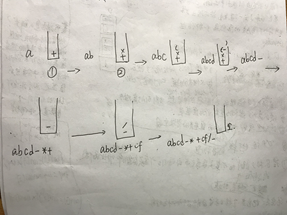中缀表达式求后缀表达式：

a+b*(c-d)-e/f

1. 从左到右将遇到的运算符入栈，遇到操作数直接将其写下来就行
2. 在将运算符入栈的时候先看看是否即将入栈的运算符优先级是否高于栈顶运算符的优先级，如果高则直接入，如果低，那么就进行出栈，直到栈顶的运算符优先级低于即将要入的运算符优先级，将弹出的运算符 跟在刚刚的操作数后面就行。
3. 如果入栈的时候运算符是右括号那么就出栈，直到出栈遇到左括号再停止出栈

三、将中缀表达式画成二叉树，然后对其进行前序和后序遍历即可

1. 先从右向左遍历中缀表达式，找到从右到左的第一个优先级最低的运算符来做二叉树的根，确定根之后那么它的左右子树基本确定，然后第二次遍历中缀表达式找第二优先级最低的运算符，然后根据刚刚的大致位置确定第二最低优先级运算符的位置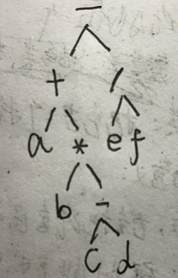展开全文• 3、从右至左扫描中缀表达式，如果是数字，就直接压入结果栈 若是运算符，则与运算符栈顶元素比较优先级：若该运算符优先级大于等于栈顶元素，则将该运算符入栈 否则栈内元素出栈并压入结果栈，再与其比较，直到该...
• 这是一个循环♻️过程 4）辅助栈的指针前移一位（这是因为当前指在右括号）， 5）若扫描完表达式之后，辅助栈还有元素，全部出栈，入到结果栈 此时，将中间结果栈的元素全部出栈，得到的就是前缀表达式
• 刚刚做完中缀表达式前缀表达式，想着试试看能不能把中缀表达式转化为前缀表达式，我自己笨想不来怎么做，然后就在网上找了很多教程，看看原理以及思路。 找了一个最笨的解决方法就是先逆序读中缀表达式，然后照着...指针 数据结构 程序设计 堆栈
• 中缀表达式前缀表达式java实现 准备知识： 前缀、中缀、后缀表达式 http://note.youdao.com/noteshare?id=c27a0ee6222c18ec60cf5bb2154b0744&sub=4CEC6E1E196B4B228839B9220F9F673C 前缀、中缀、后缀表达式和...中缀表达式 前缀表达式
• 1.1中缀表达式 普通表达式,即操作符位于操作数的中间。如''2+3*5'',''(2+3)*5''。这种表达式的特点是根据运算符的优先级不同，计算顺序不同。可以通过添加括号来改变计算的顺序，这种表达式人类理解起来没什么...python 数据结构 算法
• 中缀表达式转换成前缀表达式和后缀表达式的极其简单方法 import java.util.Arrays; import java.util.Scanner; import java.util.Stack; /* * 关于算术表达式的计算 * 中缀表达式前缀表达式求解 * ( 3 + 4 ) ...
•数据结构 算法 程序设计
• 单纯的中缀表达式会引起计算歧义，所以为了计算一般要完全括号化；或者将中缀转换为前缀或者后缀。注意：前缀和后缀没有歧义。 由中缀前、后缀的区别 不同1:优先级比较函数不同 在中缀后缀时，我们定义的...后缀表达式 前缀表达式 cpp
• 文章目录前缀表达式（波兰表达式）前缀表达式分析与介绍思路分析中缀表达式中缀表达式分析与介绍后缀表达式（逆波兰表达式）后缀表达式分析与介绍思路分析逆波兰计算器代码实现逆波兰计算器中缀表达式转换为后缀...
• 本文主要介绍中缀表达式前缀表达式算法和代码实现数据结构 算法
• 中缀表达式转前缀表达式 c++

千次阅读 2018-08-15 15:50:44
/** 北理2013年第三题  2018-8-15 13:42 输入表达式，输出相应二叉树的前序遍历结果 ...(2) 从右至左扫描中缀表达式； (3) 遇到操作数时，将其压入S2； (4) 遇到运算符时，比较其与S1栈顶运算符的优先级：  ...stack c
• 本文运用c++实现了三种表达式求值 1.前缀表达式 ：直接递归 2.后缀表达式：用栈 3.中缀表达式：转化为后缀表达式求c++ 数据结构 字符串
• 中缀表达式就是我们数学中常用的那种表达式，本文打算以中缀表达式：1 + (2 + 3) × 4 - 5 为例，分享一下前缀表达式和后缀表达式的如何形成与在计算机如何计算前后最表达式和后缀表达式 1、前缀表达式和后缀表达式...数据结构
• 这里再做一个前缀表达式的总结： 初始化两个栈：运算符栈S1和储存中间结果的栈S2； 从右至左扫描中缀表达式； 遇到操作数时，将其压入S2； 遇到运算符时，比较其与S1栈顶运算符的优先级： 4.1 如果S1为空或栈顶...java 数据结构
• 中缀表达式前缀表达式、后缀表达式 1）中缀表达式：操作运算符在操作数中间 （3+2）*4-6 2）前缀表达式：波兰式，操作运算符在操作数之前， 如-*+3246。 从右至左扫描表达式，遇到数字时，将数字压入堆栈，遇到...数据结构
• 中缀表达式：a+b 前缀表达式（波兰式）：+ab 后缀表达式（逆波兰式）：ab+ 表达式转换问题（考研经典） 一：手工转换 考研中有一类经典的问题就是表达式的转换问题，经常要把一个类型的表达式转换为另一个类型的...表达式转换
• 作完了中缀前缀，作一个归纳吧。 https://www.cnblogs.com/unixfy/p/3344550.html # coding = utf-8 class Stack: def __init__(self): self.items = [] # 是否为空 def is_empty(self): ...
• 用dev c++写的代码，附有啰里啰嗦的注释和测试样例。太简单了不好意思要分。
• (2) 从右至左扫描中缀表达式；(3) 遇到操作数时，将其压入S2；(4) 遇到运算符时，比较其与S1栈顶运算符的优先级：(4-1) 如果S1为空，或栈顶运算符为右括号“)”，则直接将此运算符入栈；(4-2) 否则，若优先级比栈顶...c语言前缀编码
• 目的将中缀表达式（即标准的表达式）转换为前缀表达式例如：1+2*3+(4*5+6)7 转换成 ++1*23+*4567转换原则：与中缀转后缀不同，前者是顺序从左到右读取每一个字符，后者是从右到左顺序读取每一个字符，然后进行反转...
• c语言实现中缀表达式转后缀表达式并求得计算结果，用顺序栈结构。 当输入者输入错误信息的时候需要报错，并说明错误的种类。
• 一、中缀表达式转后缀表达式这里的运算思路跟我代码一样，所以我就直接借鉴的别人的逆波兰表达式又称作后缀表达式，在四则混合运算的程序设计中用到。例如：1+2写成后缀表达式就是12+4+5*(3-2)的后缀表达式就是4532-......